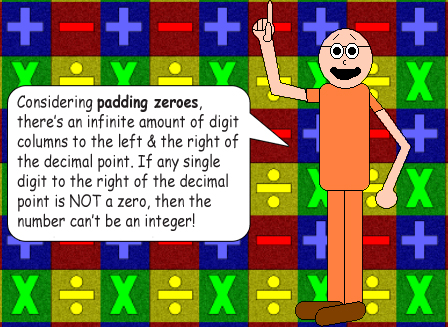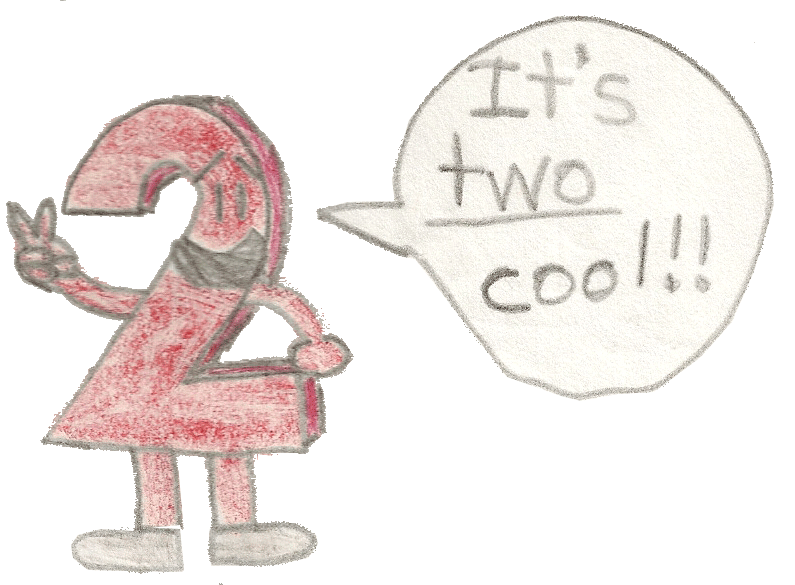# The Digits in Halves of Even Numbers

## Depending on the tens' digit of an even number, its half will either have 1 specific digit or another in the ones' column; these 2 possible digits always have a difference of 5 in absolute value!### Observe the table below:

 Digit in tens' column is… Digit in ones' column Ones' digit in even number's half Even 0 0 Odd 0 5 Even 2 1 Odd 2 6 Even 4 2 Odd 4 7 Even 6 3 Odd 6 8 Even 8 4 Odd 8 9

## Examples:

12 ÷ 2 = 6

22 ÷ 2 = 11

02 ÷ 2 = 1

Can you tell why I put that padding zero there? That's right! Zero is an even number, so the digit in the ones' column of the half is 1! If the even number has a single digit, then the tens' column digit is automatically equal to zero(0)! But let's add a digit in the hundreds' column!

102 ÷ 2 = 51

Voila! We still have the digit 1 in the ones' column of the half! Including a hundreds' column, or a thousands' column and so on won't make a difference in this math trick! The table above remains correct no matter how many digit columns you include! The trick focuses on the tens' column & the ones' column.

14 ÷ 2 = 7

64 ÷ 2 = 32

306 ÷ 2 = 153

836 ÷ 2 = 418

88 ÷ 2 = 44

158 ÷ 2 = 79

370 ÷ 2 = 185

480 ÷ 2 = 240

Look at the ones' & tens' digits in the dividends, then the ones' digit in the quotients. (All of the quotients are halves to the dividends since each dividend is divided by 2)It doesn't matter how digits the even integer has! The table above remains accurate about its half!

1,006 ÷ 2 = 503

10,006 ÷ 2 = 5,003

250,006 ÷ 2 = 125,003

175,036 ÷ 2 = 87,518

630,000,036 ÷ 2 = 315,000,018